# Formula for Uniform Accelerated Motion

Starting from this lecture, we will learn about acceleration motion in earnest.

However, it may be difficult to investigate motions with random velocity changes, so in high school physics, we investigate motions with regular velocity changes, i.e., Uniform accelerated motion.

In the previous lecture, we defined acceleration as the change in velocity per unit time.

Uniform accelerated motion is, as the name implies, motion with constant acceleration, i.e., motion in which the velocity of an object increases or decreases at a constant rate.

Since the increase (or decrease) in velocity is constant, the v-t graph for uniform accelerated motion becomes a straight line graph as shown below. Note the slope of the graph.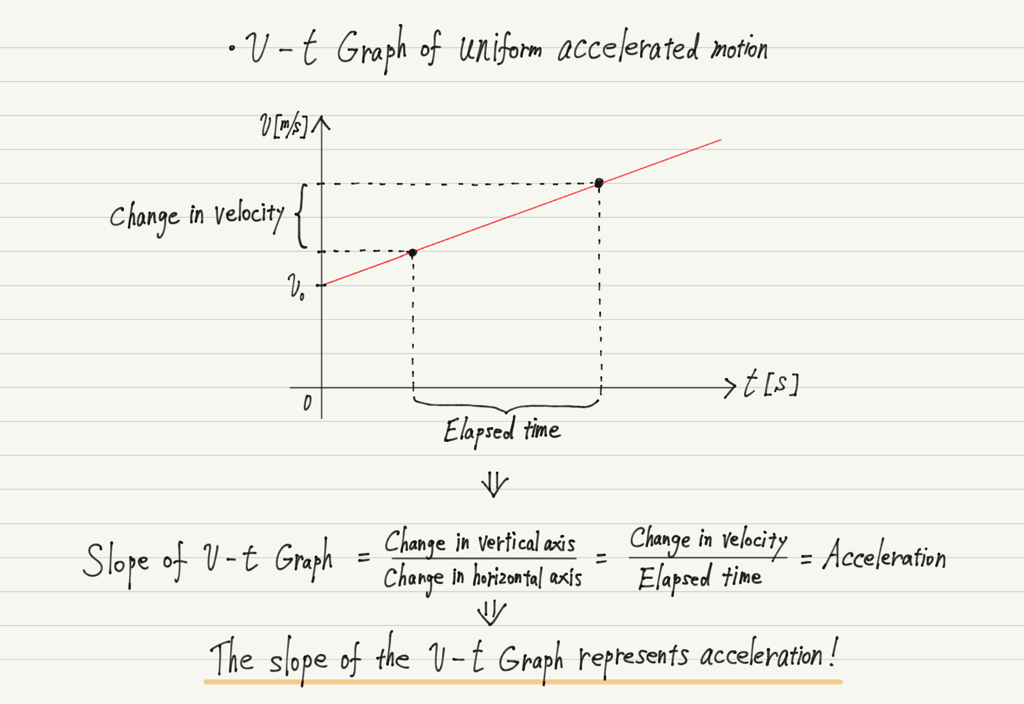## Three formulas for uniform accelerated motion

The goal of this lecture is to investigate an object in uniform accelerated motion.
Specifically, we want to find the speed and position 10 seconds after the start, or the time it takes to go 20 meters.

This may seem difficult because the velocity changes unlike in uniform linear motion, but there is a formula for obtaining these values, and I will introduce it to you!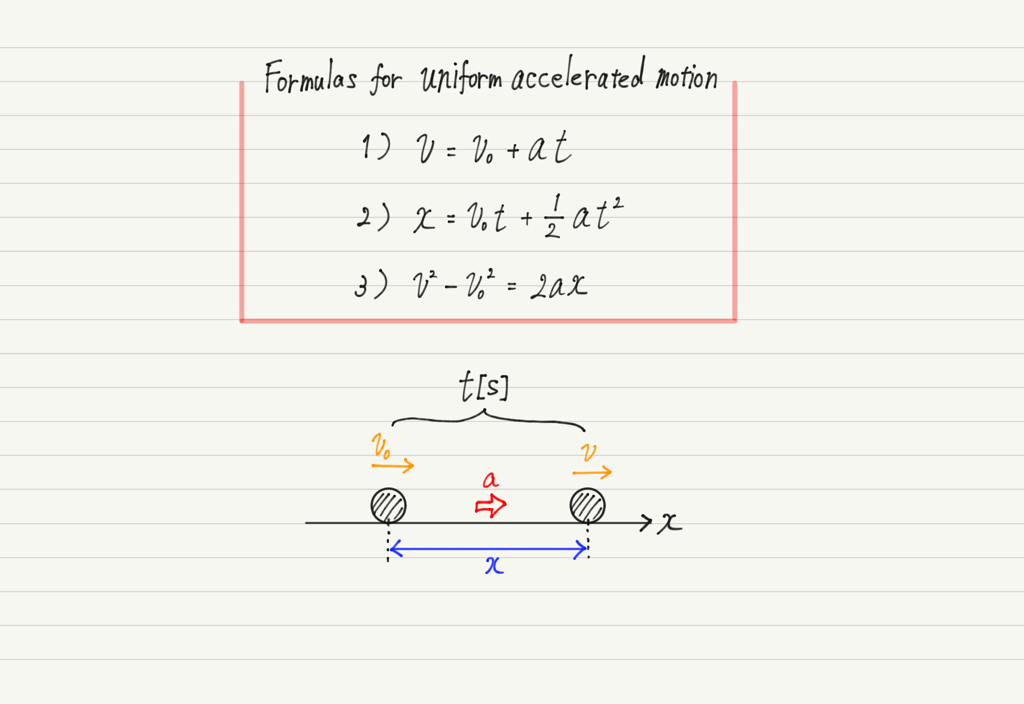It’s a hard formula to remember because it has a lot of symbols, but let’s do our best to remember it!

The derivation of these three formulas is explained below.

## Derivation of the equation for uniform accelerated motion

First, formula 1) is just a variant of the definition of acceleration from the previous lecture.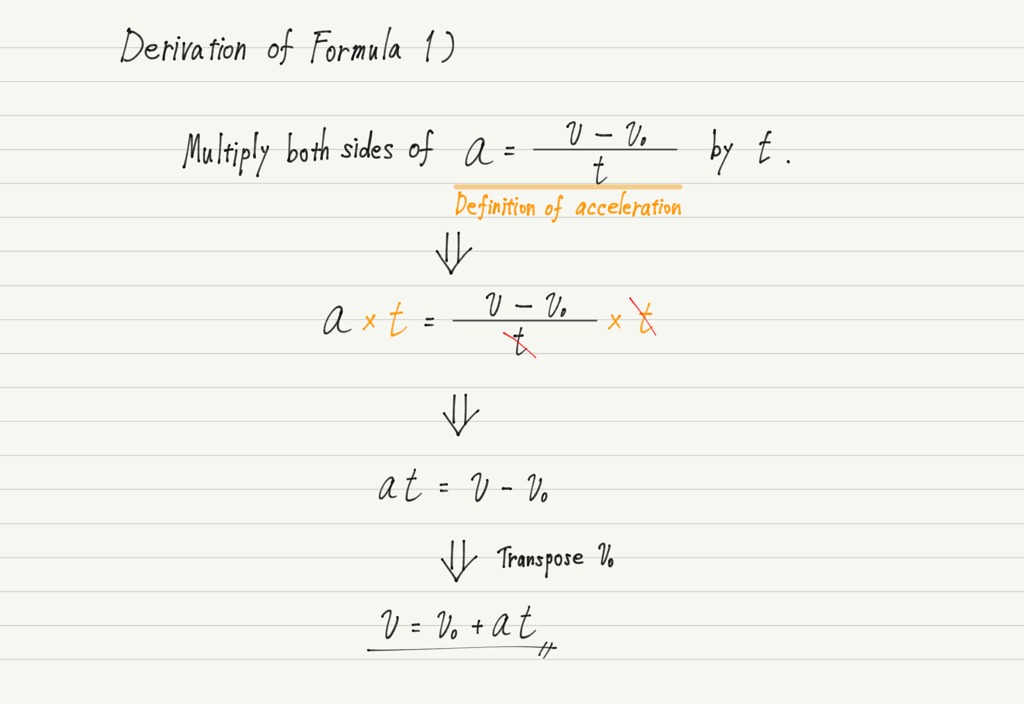Next is Formula 2). This is a formula for finding the displacement x, but it is not straightforward.

We will use the previously learned fact that the area enclosed by a v-t graph represents distance.

https://www.yukimura-physics.com/entry/dyn-f02

This “Area of v-t graph = Distance” is actually true for other than uniform linear motion!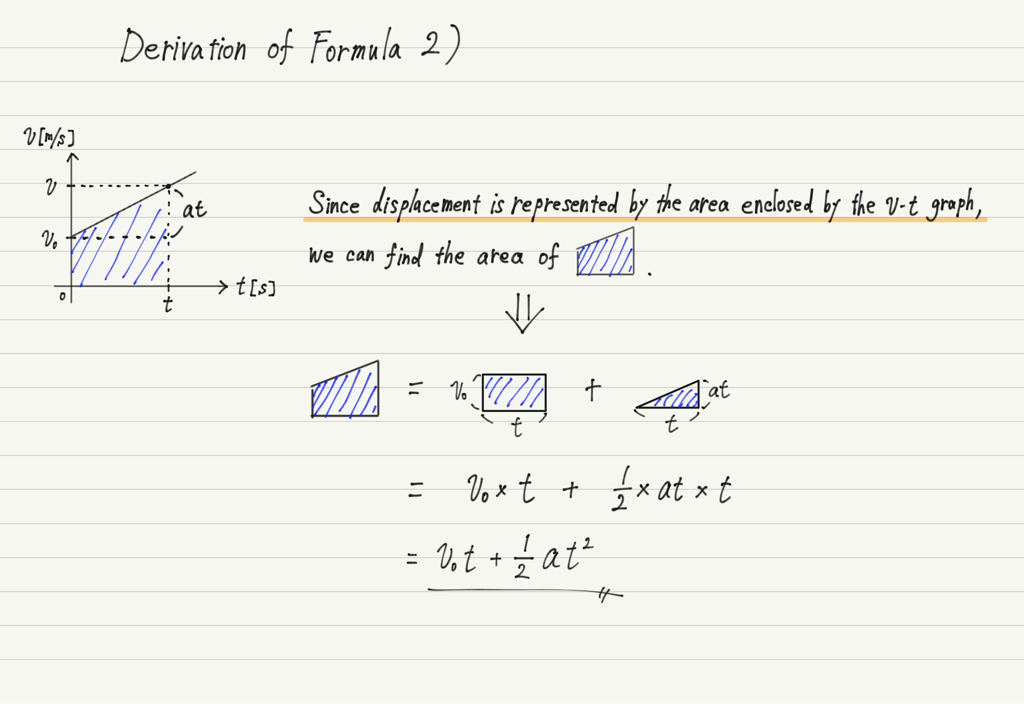Finally, formula 3) can be obtained by jointly solving formulas 1) and 2).
Specifically, transform formula 1) into the form t= … and substitute it into t in formula 2), and you are done.

In this way, the three formulas are derived. If possible, we would like you to master how to obtain the formulas, but as a practical matter, it is difficult to derive the formulas by yourself every time, so you should memorize the formulas so that you can use them right away.

## Summary of this lecture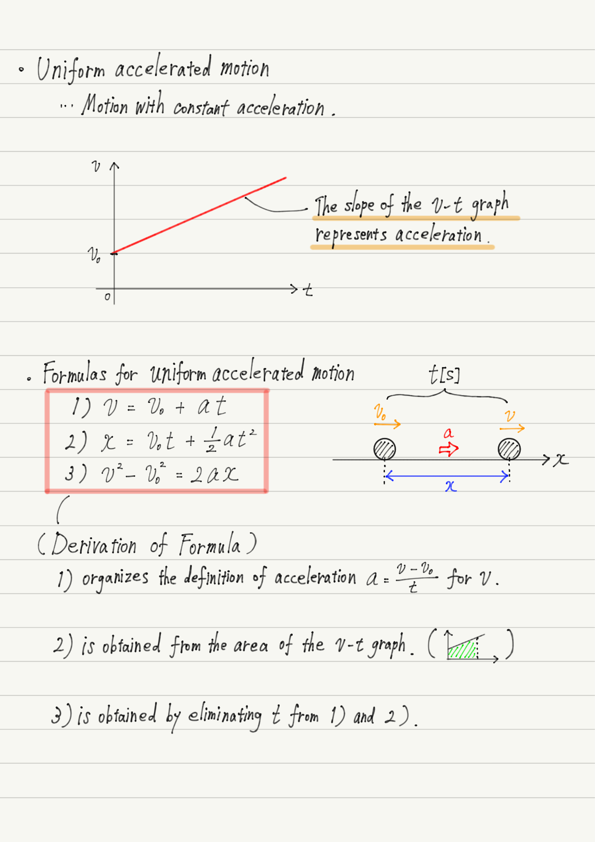## Next Time

Uniform accelerated motion will continue after the next lecture. Memorize the formulas while you still can!

https://www.yukimura-physics.com/entry/dyn-f07

error: Content is protected !!